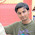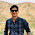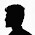C code for Least Recently Used(LRU) Algorithm

Least Recently Used (LRU):
The least recently used (LRU) policy replaces the page in memory that has
not been referenced for the longest time. By the principle of locality, this should be the page least likely to be referenced in the near future. And, in fact, the LRU policy does nearly as well as the optimal policy.

/* C program to implement least recently used algorithm */

#include<stdio.h>
#include<conio.h>

void main()
{
int page,frame,i=0,j,k=0,l=0,n,num,a,found,pf=0,x,min,ch;
char c;
FILE *fp;
clrscr();
fp=fopen("fifo.txt","r");
while(fscanf(fp,"%d%c",&a,&c)!=EOF)
page[i++]=a;
n=i;

while(1)
{
i=0,k=1,found=0;
printf("\nEnter the frame size:");
scanf("%d",&num);
for(i=0;i<num;i++)
{
frame[i]=0;
frame[i]=-1;
}

for(i=0;i<n;i++)
{
found=0;
for(j=0;j<num;j++)
if(page[i]==frame[j])
{
found=1;
x=j;
frame[x]=k++;
}
if(found==0)
{
min=n+1;
for(j=0;j<num;j++)
if(min>frame[j])
{
min=frame[j];
x=j;
}
frame[x]=page[i];
frame[x]=k++;
pf++;
}

for(l=0;l<num;l++)
{
if(frame[l]==-1)
printf("|  ");
else
printf("|%d ",frame[l]);
}
if(found==0)
printf("Page Fault\n");
else
printf("No page fault\n");
printf("\n\n");
}
printf("\Total page faults=%d",pf);
pf=0;
printf("\nPress 1 to continue:");
scanf("%d",&ch);

if(ch!=1)
break;
}
getch();
}

Input File:

7,7,4,4,2,1,4,1,5,3,2,1,4,7,6,1,9,8,0,1

//Output Of the above program:-

1.Easier way to write code of LRU in c++

#include
#include
#include
#include

using namespace std;

int main()
{
int p={2,3,2,1,5,2,4,5,3,2,5,2},f=3,frame={-1,-1,-1};
int arr={0,0,0},flag=0,pos,counter=1,m;

for(int i=0;i<12;i++)
{ pos=0;
for(int j=0;j<3;j++)
{
if(p[i]==frame[j])
{
flag=1;
pos=j;
}
}

if(flag==1)
{
arr[pos]=counter;

}

if(flag==0)
{
m=arr;

for(int j=0;j<3;j++)

{

if(m>arr[j])
{
m=arr[j];
pos=j;
}
}

frame[pos]=p[i];
arr[pos]=counter;
}

counter++;

cout<<frame<<frame<<frame<<"\t\t";
cout<<arr<<arr<<arr<<"\n";
flag=0;

}
return 0;

}

2.This comment has been removed by the author.

3.sir please mail me a openGL code for the same to sheetalshirahattijain@gmail.com